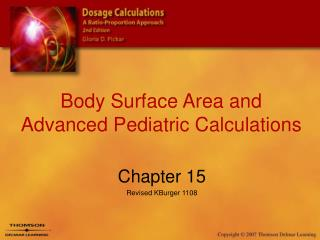DownloadDownload PresentationBody Surface Area and Advanced Pediatric Calculations

# Body Surface Area and Advanced Pediatric Calculations

Download Presentation## Body Surface Area and Advanced Pediatric Calculations

- - - - - - - - - - - - - - - - - - - - - - - - - - - E N D - - - - - - - - - - - - - - - - - - - - - - - - - - -
##### Presentation Transcript

1. Body Surface Area and Advanced Pediatric Calculations Chapter 15 Revised KBurger 1108

2. BSA (m2) Calculation • To calculate Body Surface Area (BSA) in m2 based on metric measurement of height and weight: • BSA (m2) =

3. BSA (m2) Calculation • To calculate BSA in m2 based on household measurement of height and weight: • BSA (m2) =

4. BSA (m2) Calculation • Calculate in Metric • BSA of a child whose height is 105 cm (42 in) and weight is 31.8 kg (70 lb).

5. BSA (m2) Calculation

6. BSA (m2) Calculation • Calculate in Household • BSA of a child whose height is 105 cm (42 in) and weight is 31.8 kg (70 lb).

7. BSA (m2) Calculation

8. Verifying Safe Dosages: BSA Method • Verify safe pediatric dosage based on BSA: • Determine BSA in m2. • Calculate the safe dosage based on BSA:mg per m2× m2 = X mg • Compare ordered dosage to recommended dosage • Decide if the dosage is safe.

9. Verifying Safe Dosages: BSA Method • If dosage is safe • Calculate amount to give and administer dose • If dosage seems unsafe, consult with ordering practitioner before administering

10. Verifying Safe Dosages: BSA Method • Note: Recommended dosage may specify: • mg per m2 • Mcg per m2 • g per m2 • Units per m2 • M units per m2 • mE q per m2

11. Calculation of Safe Dosage by BSA • A child is 126 cm tall and weighs 23 kg. • Drug order reads: Oncovin 1.8 mg IV at 10 A.M. • Is this dosage safe? • Recommended dosage is 2 mg per m2 .

12. Calculation of Safe Dosage by BSA

13. Calculation of Safe Dosage by BSA • Determine BSA • Calculate recommended dosage Mg per m2× m2 = 2 mg per m2 × 0.9 m2 = 1.8 mg

14. Calculation of Safe Dosage by BSA • 3. Decide if the dosage is safe • Dosage ordered is 1.8 mg and 1.8 mg is amount recommended by BSA • 4. Calculate one dose

15. Calculation of Safe Dosage by BSA • Step 1. Convert • No conversion is necessary. • Step 2. Think • Give more than 1 mL and less than 2 mL

16. Calculation of Safe Dosage by BSA • Step 3. Calculate • Use ratio-proportion: X = 1.8 mL

17. See page 380 in Pickar BSA Nomogram • Chart that estimates BSA by plotting height and weight. • Find height and weight on columns and draw a straight line between them • Point where crosses the SA column = BSA • NOTE: incremental spaces are not consistent. Take care to read numbers and calibration values correctly.

18. Total Daily IV Fluid for Children • Use this formula to calculate the daily rate of pediatric maintenance IV fluids: • 100 mL per kg per day for the first 10 kg of body weight • 50 mL per kg per day for the next 10 kg of body weight • 20 mL per kg per day for each kg above 20 kg of body weight

19. Total Daily IV Fluid for Children • Child who weighs 24 kg • 100 mL per kg per day × 10 kg = 1000 mL per day (for first 10 kg) • 50 mL per kg per day × 10 kg = 500 mL per day (for next 10 kg) • 20 mL per kg per day × 4 kg = 80 mL per day (for remaining 4 kg) TOTAL 1580mL per day

20. Total Daily IV Fluid for ChildrenCalculating hourly IV flow rate 1000 mL per day + 80 mL per day + 500 mL per day = 1580 mL per day